Tamilnadu State Board New Syllabus Samacheer Kalvi 8th Maths Guide Pdf Chapter 5 Geometry Ex 5.3 Text Book Back Questions and Answers, Notes.

## Tamilnadu Samacheer Kalvi 8th Maths Solutions Chapter 5 Geometry Ex 5.3

Question 1.
In the figure, given that ∠1 = ∠2 and ∠3 ≡ ∠4. Prove that ∆ MUG ≡ ∆TUB.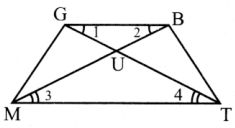Statements Reasons 1. In △MUG and △TUG Mu = TU ∠3 = ∠4, opposite sides of equal angles 2. UG = UB ∠1 = ∠2 Side opposite to equal angles are equal 3. ∠GUM = ∠BUT Vertically opposite angle 4. ∆MUG ≡  ∆TUG SAS criteria By 1,2 and 3Question 2.
From the figure, prove that ∆SUN ~ ∆RAY.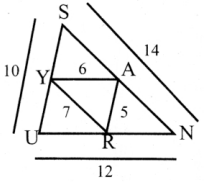Proof: from the ∆ SUN and ∆RAY
SU = 10
UN = 12
SN = 14
RA = 5
AY = 6
RY = 7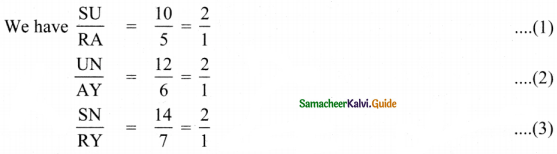From (1), (2) and (3) we have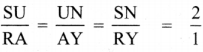The sides are proportional
∴ ∆SUN ~ ∆RAYQuestion 3.
The height of a tower is measured by a mirror on the ground at R by which the top of the tower’s reflection is seen. Find the height of the tower. If ∆PQR ~ ∆STR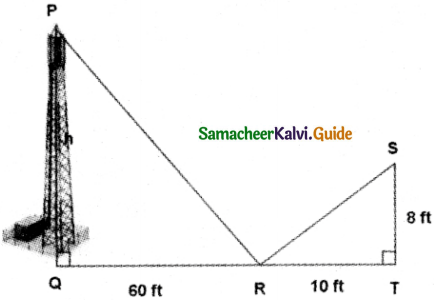The image and its reflection make similar shapes
∴ ∆PQR ~ ∆STR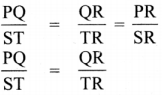⇒ $$\frac{h}{8}=\frac{60}{10}$$
h = $$\frac{60}{10}$$ × 8
= 48 feet
∴ Height of the tower = 48 feet.Question 4.
Find the length of the support cable required to support the tower with the floor.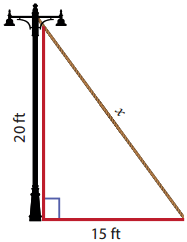From the figure, by Pythagoras theorem,
x2 = 202 + 152
= 400 + 225 = 625
x2 = 252 ⇒ x = 25ft.
∴ The length of the support cable required to support the tower with the floor is 25ft.Question 5.
Rithika buys an LED TV which has a 25 inches screen. If its height is 7 inches, how wide is the screen? Her TV cabinet is 20 inches wide. Will the TV fit into the cabinet? Give reason.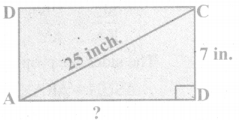Take the sides of a right angled triangle ∆ABC as
a = 7 inches
b = 25 inches
c = ?
By Pythagoras theorem,
b2 = a2 + c2
252 = 72 + c2
⇒ c2 = 252 – 72 = 625 – 49 = 576
∴ c2 = 242
⇒ c = 24 inches
∴ Width of TV cabinet is 20 inches which is lesser than the width of the screen ie.24 inches.
∴ The TV will not fit into the cabinet.Challenging Problems

Question 6.
In the figure, ∠TMA ≡∠IAM and ∠TAM ≡ ∠IMA. P is the midpoint of MI and N is the midpoint of AI. Prove that Δ PIN ~ Δ ATM.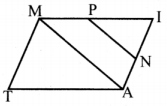proof:Question 7.
In the figure, if ∠FEG ≡ ∠1 then, prove that DG2 = DE.DF.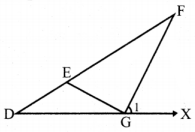Proof: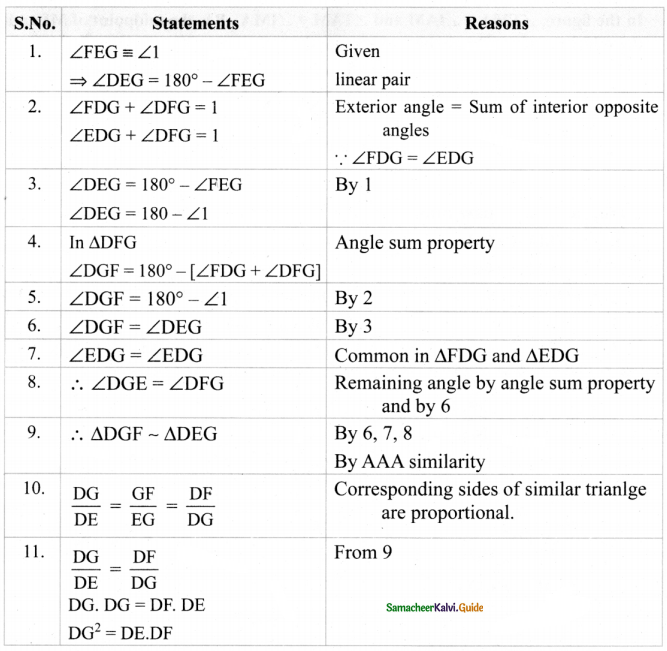Question 8.
The diagonals of the rhombus is 12 cm and 16 cm. Find its perimeter. (Hint: the diagonals of rhombus bisect each other at right angles).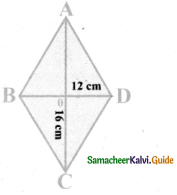Here AO = CO = 8cm
BO = DO = 6cm
(∴the diagonals of rhombus bisect each other at right angles)
∴ In ∆ AOB, AB2 = AO2 + OB2
= 82 + 62 = 64 + 36
= 100 = 102
∴ AB = 10
Since it is a rhombus, all the four sides are equal.
AB = BC = CD = DA
∴ Its Perimeter = 10 + 10 + 10 + 10 = 40 cmQuestion 9.
In the figure, find AR.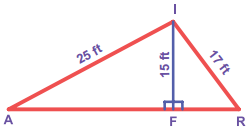∆ AFI, ∆ FRI are right triangles.
By Pythagoras theorem,
AF2 = AI2 – FI2
= 252 – 152
= 625 – 225 = 400 = 202
∴ AF = 20ft.
FR2 = RI2 – FI2
= 172 – 152 = 289 – 225 = 64 = 82
FR = 8ft.
∴ AR = AF + FR
= 20 + 8 = 28 ft.Question 10.
In ∆DEF, DN, EO, FM are medians and point P is the centroid. Find the following.
(i) IF DE = 44, then DM = ?
(ii) IFPD=12, then PN= ?
(iii) IfDO = 8, then PD = ?
(iv) IF 0E = 36 then EP = ?Given DN, EO, FM are medians.
∴ FN = EN
DO = FO
EM = DM

(i) If DE = 44,then
DM = $$\frac{44}{2}$$ = 22
DM = 22

(ii) If PD = 12,PN = ?
$$\frac{P D}{P N}=\frac{2}{1}$$
$$\frac{12}{\mathrm{PN}}=\frac{2}{1}$$ ⇒ PN = $$\frac{12}{2}$$ = 6.
PN = 6(iii) If DO = 8, then
FD = DO + OF
= 8 + 8
FD = 16

(iv) If OE = 36PE = 24